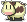3,632 PagesThis article is a stub. You can help The Harvest Moon Wiki by expanding it.

## Crops

Blueprint Materials
Gravel Field Soil (x8)

Regular Field

Soil (x8) + Fodder (x5)

Soil Field

Soil (x8) + Fodder (x5) + Fertilizer (x5)

Soil (x8) + Small Material Stone (x5)
Beehive  Small Lumber (x8) + Honeycomb (x5)
Mushroom Log Branch (x5)

## Buildings

Blueprint Materials Sell Cost
Barn  Material Stone (x5) + Small Lumber (x30) + Fodder (x5)
Coop  Small Material Stone (x5) + Small Lumber (x20) + Mint (x5) 300
Pet House  Material Stone (x5) + Lumber (x20) + Brick (x5) + Moondrop Flower (x5)
Maker Shed Material Stone (x20) + Lumber (x20) + Brick (x3) + Amethyst (x1)
Supply Shed Small Material Stone (x10) + Small Lumber (x20)
Fishing Shed  Small Material Stone (x20) + Lumber (x20) + Glass Stone (x5)

## Special

Blueprint Materials
Upgraded House Material Stone (x30) + Lumber (x30) + Glittering Stone (x2) + Brick (x5) + Glass Stone (x5)
Farm Bell Bell (x1) + Silver (x3) + Jade (x5)
Watering Spot Small Material Stone (x5)
Chicken Feeding Area Small Material Stone (x5) + Small Lumber (x2) + Soil (x3)
Gardening Space  Small Rock (x8) + Small Material Stone (x15)

## Makers

Blueprint Materials
Fermenter Small Material Stone (x10) + Bottle (x5) + Silver (x2) + Adamantite (x1)
Cloth Maker Small Material Stone (x10) + Bottle (x5) + Copper (x2) + Clippers (x3)
Seed Maker Small Material Stone (x10) + Bottle (x5) + Copper (x2) + Fertilizer (x3)
Medicine Maker Black Material Stone (x10) + Scrap Metal (x50) + Jade (x3) + Flourite (x3) + Topaz (x3)
Miller  Material Stone (x10) + Bottle (x5) + Sandrose (x3) + Iron Hammer (x1) + Walnut (x10)

Community content is available under CC-BY-SA unless otherwise noted.